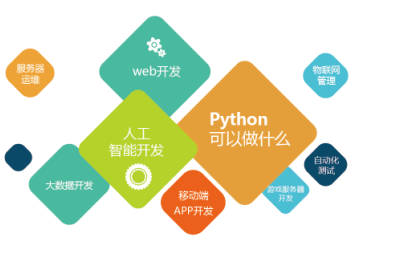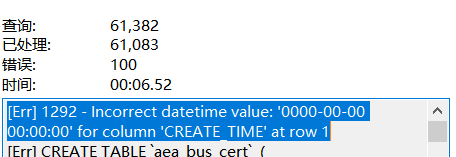Python 编程开发 实用经验和技巧

# 一、小数保留指定位小数

## 1.%f 方法

``````f = 1.23456
f1 = '%.4f' % f
f2 = '%.2f' % f
print(f1,type(f1))
print(f2,type(f2))``````

``````1.2346 <class 'str'>
1.23 <class 'str'>``````

## 2.format函数

``````f = 1.23456
f1 = format(f,'.4f')
f2 = format(f,'.2f')
print(f1,type(f1))
print(f2,type(f2))``````

``````1.2346 <class 'str'>
1.23 <class 'str'>``````

## 3.round()函数

``````a = 1.23456
b = 2.355
c = 3.5
d = 2.5
a1 = round(a, 3)
b1 = round(b, 2)
c1 = round(c)
d1 = round(d)
print(a1,type(a1))
print(b1,type(b1))
print(c1,type(c1))
print(d1,type(d1))``````

``````1.235 <class 'float'>
2.35 <class 'float'>
4 <class 'int'>
2 <class 'int'>``````

## 4.直接截断

``````a = int(1.23456 * 1000) / 1000
print(a)``````

# 二、判断变量的数据类型

## 1.type():

``````a = 1.23
print(type(a))``````

## 2.isinstance()

``````a = 1.23
tp = isinstance(a,float)
print(tp)``````

## 3.class.name属性

``````num = 1.23

print(num.__class__.__name__)``````

``float``

# 三、Python中的类方法(@classmethod)

python做面向对象编程的时候，经常需要使用`classmethod`类方法，这并不是类中的方法。 类方法也算一种实用的技巧，简单描述之：“类方法让类模板具有记忆力”。 类模板就是我们所定义的类。在普通情况下，不使用类方法对类进行实例化，类本身是不具有记忆性的。只是当一个静态模板被套用多次而已。如果我们想让类在每一次实例化之后，都能记载一些记忆，会对很多操作很有用。

``````class Man:
id = 0 # 类变量
def __init__(self, name):
self.name = name
self.id = self.id_number()

@classmethod
def id_number(cls):
cls.id += 1
return cls.id

a = Man('A')
print(a.id)
b = Man('B')
print(b.id)``````

``````1
2``````

# 四、str.format与制表符\t设置中文对齐

str.format对字符串进行格式化，`{:<x}`的语法表示左对齐，`{:>x}`为右对齐，`{:^x}`为居中），少于x位自动补齐（默认为空格补齐），但是对于中文字符并不能很好地支持，所以会导致有多行中文字符串时出现不能对齐的现象，需要考虑到字符串所占长度并将中文字符串进行编码后再计算。

``````#name是包含中文的字符串，22是整个字符串一行的总长度，一般要顾及测试才能得到，\t后的x是一标记字符，可换为别的所需的字符串
print('[{string:<{len}}\tx'.format(string=string+']',len=22-len(string.encode('GBK'))+len(string)))``````

# 五、datetime模块timedelta类的使用

timedelta对象表示两个不同时间之间的差值， 这个差值的单位可以是：天、秒、微秒、毫秒、分钟、小时、周。 如果使用time模块对时间进行算术运算，只能将字符串格式的时间和struct_time格式的时间对象先转换为时间戳格式，然后对该时间戳加上或减去n秒，最后再转换回struct_time格式或字符串格式，这显然很不方便。而datetime模块提供的timedelta类可以让我们很方面的对`datetime.date`, `datetime.time``datetime.datetime`对象做算术运算，且两个时间之间的差值单位也更加容易控制。 datetime.timedelta类的定义：

``class datetime.timedelta(days=0, seconds=0, microseconds=0, milliseconds=0, hours=0, weeks=0)``

• 1毫秒转换为1000微秒
• 1分钟转换为60秒
• 1小时转换为3600秒
• 1周转换为7天

• microseconds : [0, 999999]
• seconds : [0, 86399]
• days : [-999999999, 999999999]

datetime.datetime.now() 返回当前本地时间（datetime.datetime对象实例）
datetime.datetime.fromtimestamp(timestamp) 返回指定时间戳对应的时间（datetime.datetime对象实例）
datetime.timedelta() 返回一个时间间隔对象，可以直接与datetime.datetime对象做加减操作
``````>>> import datetime
>>>
>>> datetime.timedelta(365).total_seconds() # 一年包含的总秒数
31536000.0
>>> dt = datetime.datetime.now()
>>> dt + datetime.timedelta(3) # 3天后
datetime.datetime(2020, 1, 22, 11, 17, 0, 214877)
>>> dt + datetime.timedelta(-3) # 3天前
datetime.datetime(2020, 1, 16, 11, 17, 0, 214877)
>>> dt + datetime.timedelta(hours=3) # 3小时后
datetime.datetime(2020, 1, 19, 14, 17, 0, 214877)
>>> dt + datetime.timedelta(hours=-3) # 3小时前
datetime.datetime(2020, 1, 19, 8, 17, 0, 214877)
>>> dt + datetime.timedelta(hours=3, seconds=30) # 3小时30秒后
datetime.datetime(2020, 1, 19, 14, 17, 30, 214877)``````

# 六、获取抛出的异常具体信息

``````def get_exception_info():
try:
s = 2 / 0
except Exception as e:
print('异常内容：', e.args)
print('异常行数：', e.__traceback__.tb_lineno)
print('异常文件：', e.__traceback__.tb_frame.f_globals["__file__"])

get_exception_info()``````

``````异常内容： division by zero

# 七、使用BeautifulSoup库去掉字符串中的HTML标签

``````text = """<div>
<h1>Title</h1>
<p>A long text........ </p>
</div>"""``````

``````from bs4 import BeautifulSoup

text = """<div>
<h1>Title</h1>
<p>A long text........ </p>
</div>"""

clean_text = BeautifulSoup(text, "lxml").text
print(clean_text)``````

``````Title
A long text........
``````浅梦一笑
4个月前blmius
1年前
MySQL:[Err] 1292 - Incorrect datetime value: ‘0000-00-00 00:00:00‘ for column ‘CREATE_TIME‘ at row 1CuterCorley
1年前
Web前端开发 HTML设计 经验与技巧总结Stella981
1年前
KVM调整cpu和内存Wesley13
1年前
4castStella981
1年前
Python之time模块的时间戳、时间字符串格式化与转换
Python处理时间和时间戳的内置模块就有time，和datetime两个，本文先说time模块。关于时间戳的几个概念时间戳，根据1970年1月1日00:00:00开始按秒计算的偏移量。时间元组（struct_time），包含9个元素。 time.struct_time(tm_yWesley13
1年前
MySQL查询按照指定规则排序
1.按照指定(单个)字段排序selectfromtable_nameorderiddesc;2.按照指定(多个)字段排序selectfromtable_nameorderiddesc,statusdesc;3.按照指定字段和规则排序selecWesley13
1年前
Java日期时间API系列36
十二时辰，古代劳动人民把一昼夜划分成十二个时段，每一个时段叫一个时辰。二十四小时和十二时辰对照表：时辰时间24时制子时深夜11：00凌晨01：0023：0001：00丑时上午01：00上午03：0001：0003：00寅时上午03：00上午0Stella981
1年前
Angular material matWesley13
1年前
MySQL部分从库上面因为大量的临时表tmp_table造成慢查询helloworld_34035044
7个月前

​在我们用皕杰报表工具设计填报报表时，如何在新增行里自动增加id呢？能新增整数排序id吗？目前可以在新增行里自动增加id，但只能用uuid函数增加UUID编码，不能新增整数排序id。uuid函数说明：获取一个UUID，可以在填报表中用来创建数据ID语法：uuid()或uuid(sep)参数说明：sep布尔值，生成的uuid中是否包含分隔符''，缺省为CuterCorley
Lv1

108

0

2